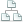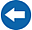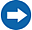Home >Tips >Excel 2016 from Scratch >MultiplicationSite map

# Excel 2016 from Scratch Exercise Multiplication

## An Excel tutorial by Peter KalmstromIn this demo in the Excel 2016 from Scratch series, Peter Kalmstrom shows how to create a formula that multiplies the value of one cell with the value of another cell.

As an example Peter uses different articles, which price should be calculated by weight and price per kilo.

### Exercise

If you want to try the exercise yourself, please download the Excel file Peter uses in the demo!

### Content

This is what Peter shows in the demo below:

• How to multiply the values of two cells.
• How to check of the formula works as it should.
• How to use Ctrl + z to undo.
• How to fill new cells with the same kind of formula.
• How to autosum all calculated values.
• How to add a currency format to cells.
• How to format a cell so that it has a border at the bottom.
• How to add a percentage sign to a number.
Peter uses Excel 2016 for his demo, but the Excel basics are the same for earlier versions of Excel.